Coefficient of Variation

Chapter 13 Class 11 Statistics
Serial order wise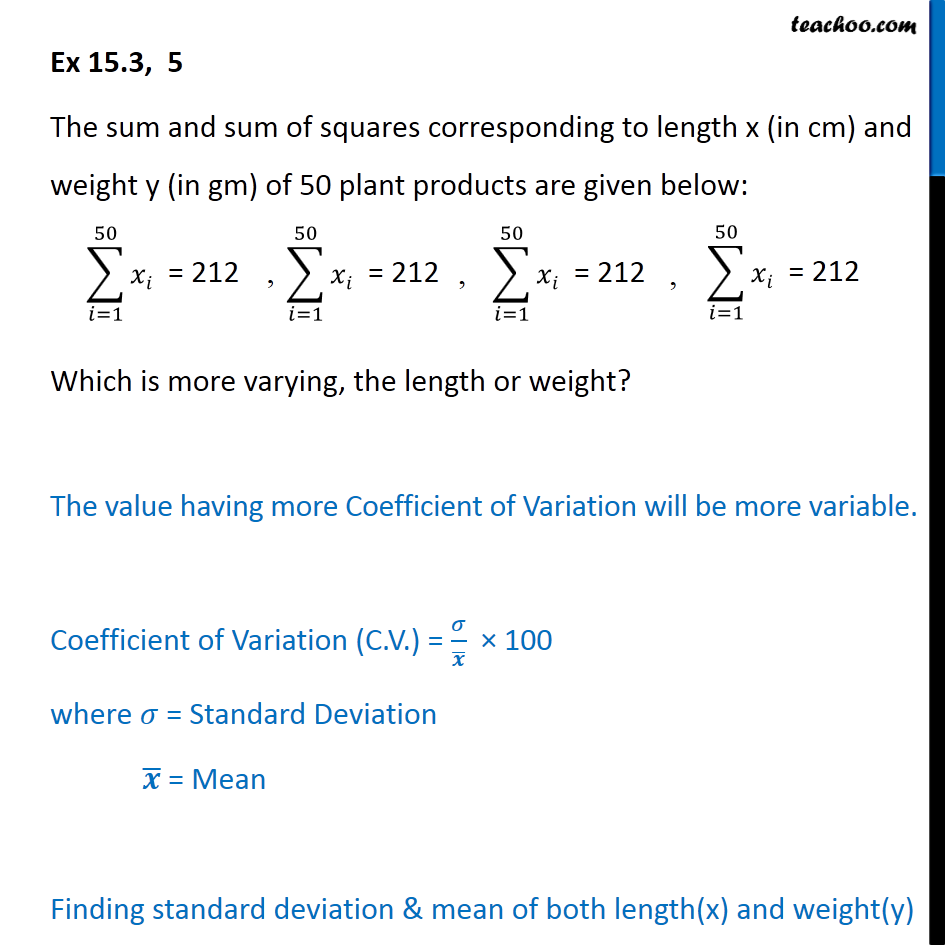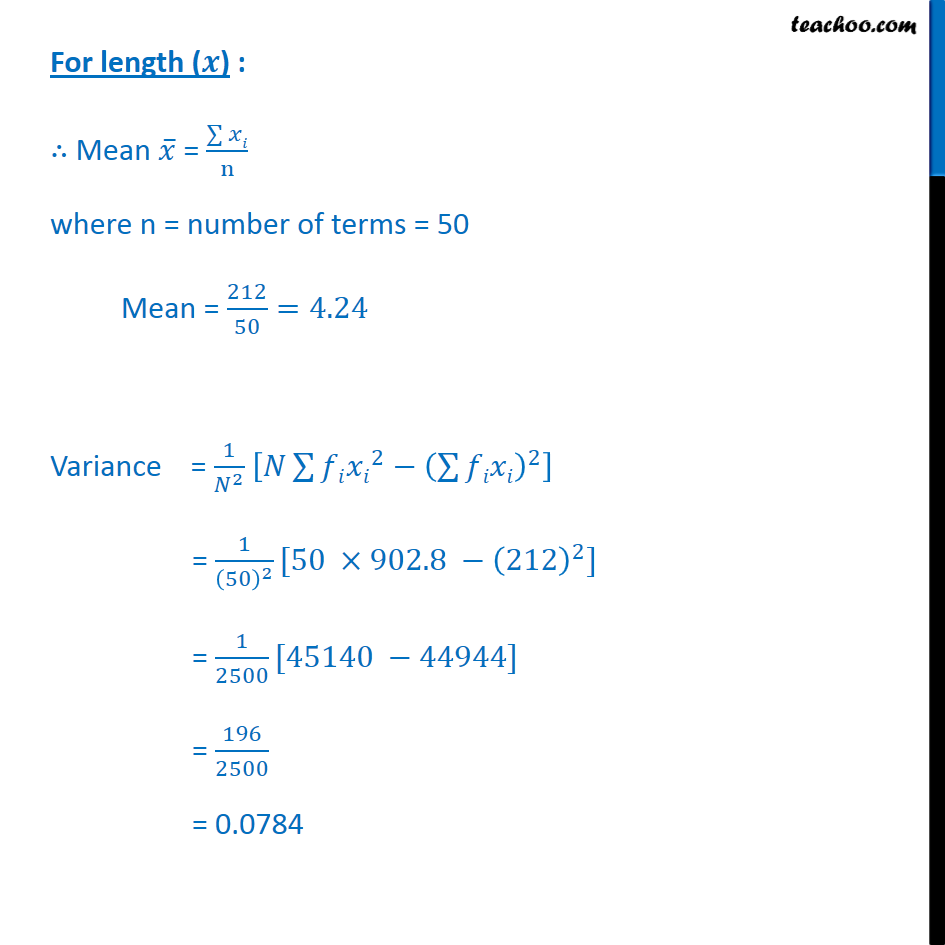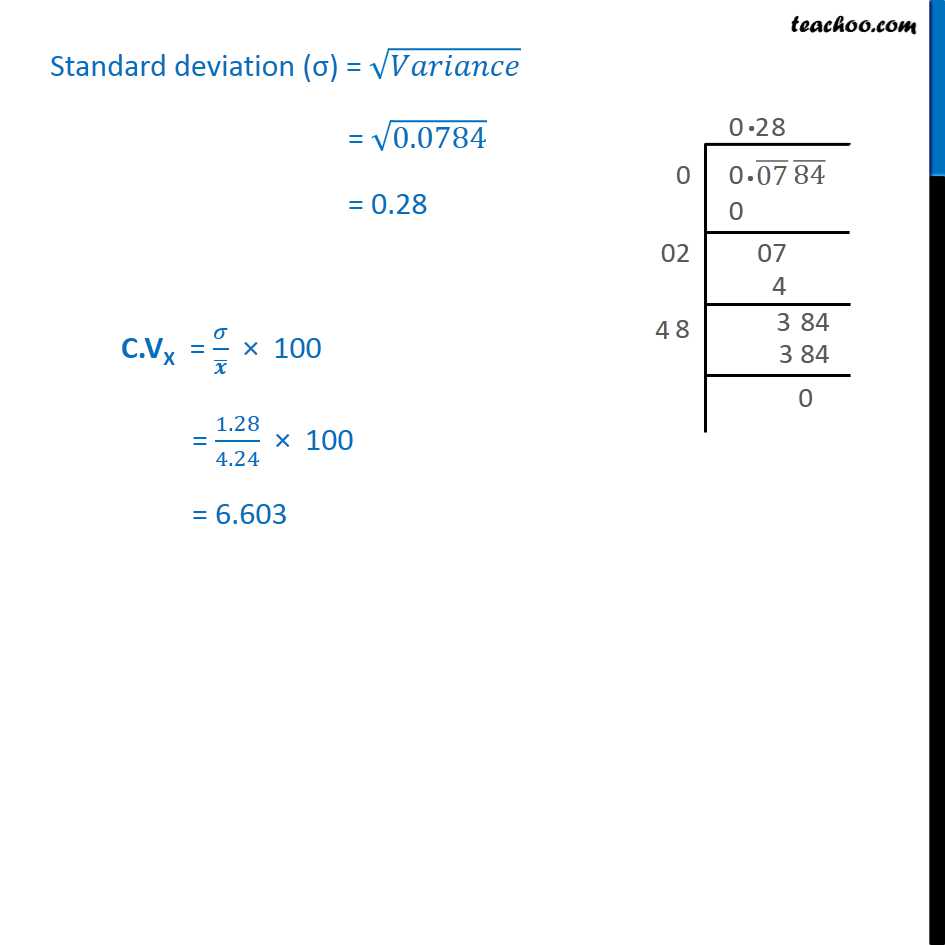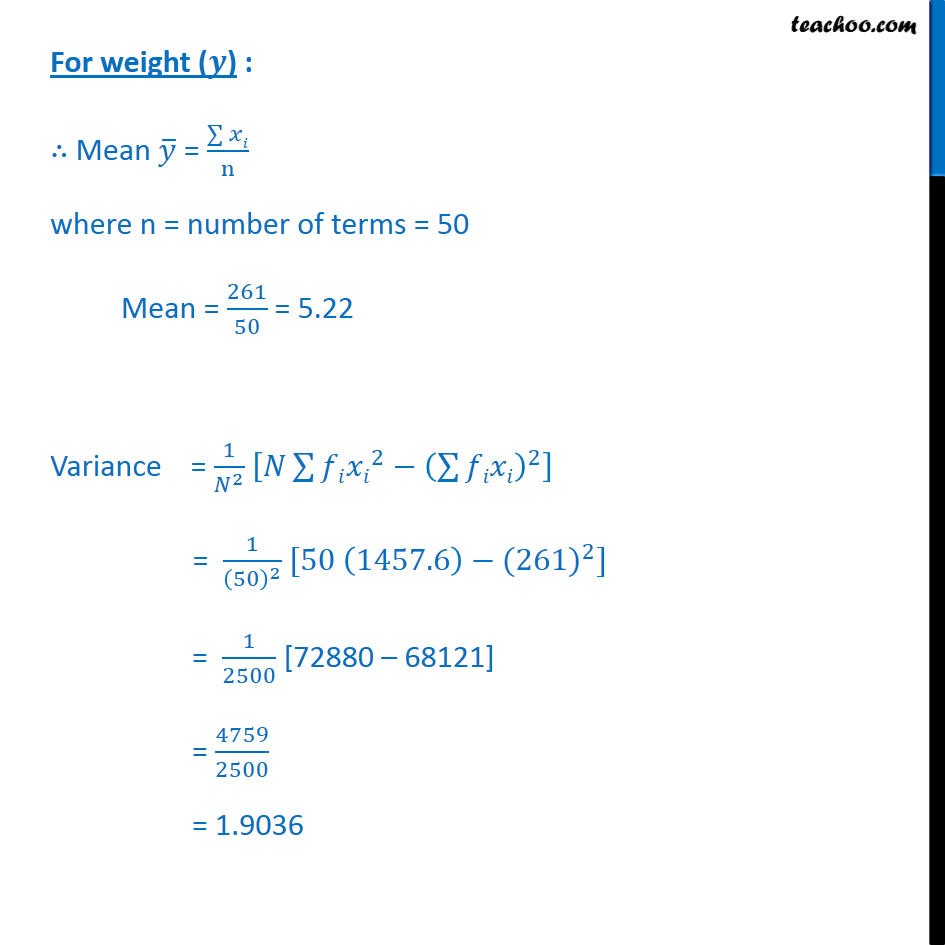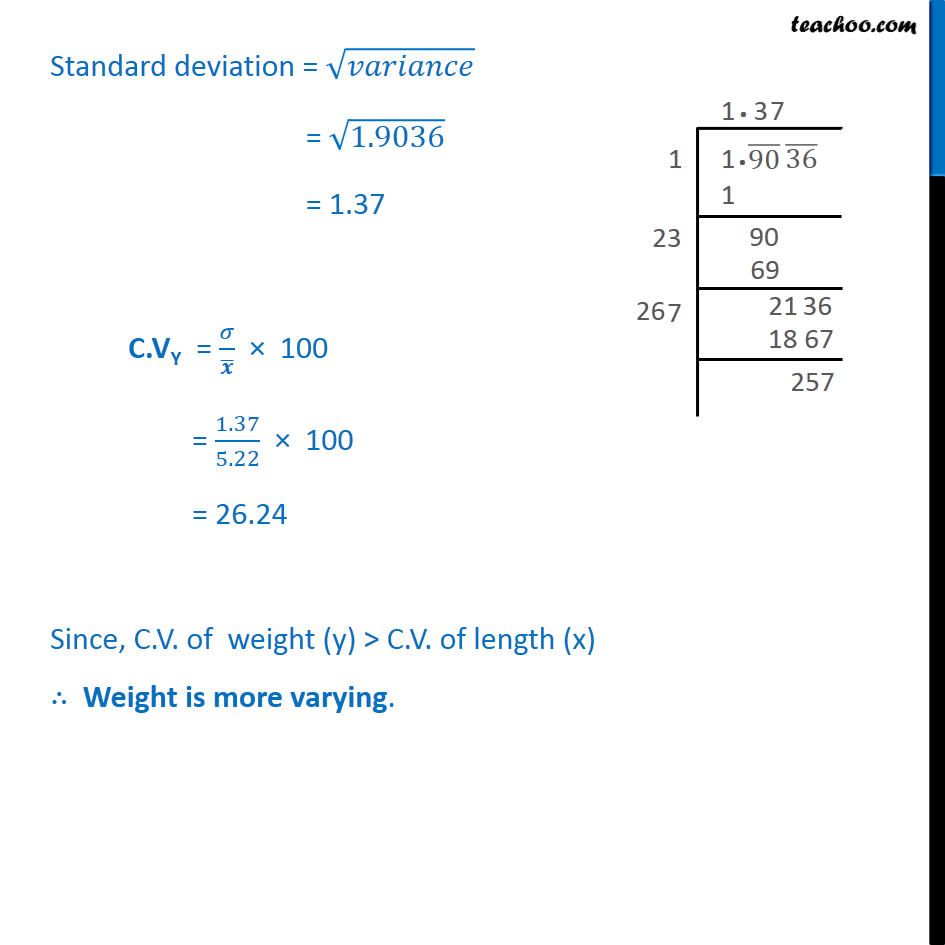Learn in your speed, with individual attention - Teachoo Maths 1-on-1 Class

### Transcript

Question 5 The sum and sum of squares corresponding to length x (in cm) and weight y (in gm) of 50 plant products are given below: Which is more varying, the length or weight? The value having more Coefficient of Variation will be more variable. Coefficient of Variation (C.V.) = 𝜎/𝒙 ̅ × 100 where 𝜎 = Standard Deviation 𝒙 ̅ = Mean Finding standard deviation & mean of both length(x) and weight(y) For length (𝒙) : ∴ Mean 𝑥 ̅ = (∑▒𝑥𝑖)/n where n = number of terms = 50 Mean = 212/50=4.24 Variance = 1/𝑁^2 [𝑁∑▒〖𝑓𝑖〖𝑥𝑖〗^2 〗−(∑▒𝑓𝑖𝑥𝑖)^2 ] = 1/(50)^2 [50 ×902.8 −(212)^2] = 1/2500[45140 −44944] = 196/2500 = 0.0784 Standard deviation ("σ") = √𝑉𝑎𝑟𝑖𝑎𝑛𝑐𝑒 = √0.0784 = 0.28 C.VX = 𝜎/𝒙 ̅ × 100 = 1.28/4.24 × 100 = 6.603 For weight (𝒚) : ∴ Mean 𝑦 ̅ = (∑▒𝑥𝑖)/n where n = number of terms = 50 Mean = 261/50 = 5.22 Variance = 1/𝑁^2 [𝑁∑▒〖𝑓𝑖〖𝑥𝑖〗^2 〗−(∑▒𝑓𝑖𝑥𝑖)^2 ] = 1/(50)^2 [50 (1457.6)−(〖261)〗^2] = 1/2500 [72880 – 68121] = 4759/2500 = 1.9036 Standard deviation = √𝑣𝑎𝑟𝑖𝑎𝑛𝑐𝑒 = √1.9036 = 1.37 C.VY = 𝜎/𝒙 ̅ × 100 = 1.37/5.22 × 100 = 26.24 Since, C.V. of weight (y) > C.V. of length (x) ∴ Weight is more varying.